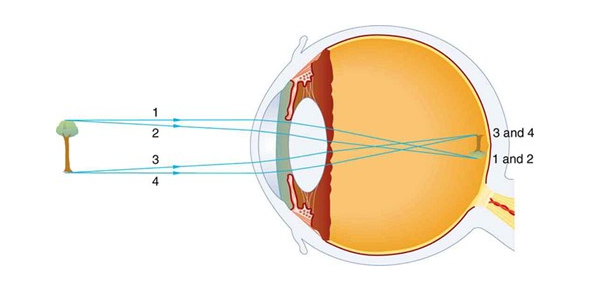# Ophthalmic Optics Mt 2

113 Questions | Total Attempts: 915Settings.

• 1.
Reflection is when light rays are redirected in a different direction, usually with a loss in amplitude
• A.

True

• B.

False

• 2.
What is refraction based on?
• A.

Wavelength of light

• B.

Index of the material

• C.

Frequency of light

• 3.
As n _____, the speed of light _______.
• A.

Decreases, decreases

• B.

Increases, increases

• C.

Increases, decreases

• 4.
The slower the light passes through a lens, the ____ it gets refracted.
• A.

Less

• B.

More

• C.

No change

• 5.
A prism will bend light rays towards its ________.
• 6.
A prism will shift an image towards its ______.
• 7.
The prism diopter is the unite for prisms and it represents:
• A.

The angle of deviation in degrees

• B.

The angle of the apical angle

• C.

The amount of image displacement

• 8.
An angle of 1 radian results in an arc with an equal length to the _______.
• 9.
• A.

360deg/2pi

• B.

57deg

• C.

360 deg

• D.

2 of the above

• 10.
1 prism diopter = ______ radians
• 11.
1 prism diopter = _____ degrees
• A.

0.57

• B.

1.57

• C.

57

• 12.
Describe in words what a prism diopter is.
• 13.
How much power does a prism have if it is able to displace an image by 5cm at a viewing distance of 0.5m?
• A.

10 cm

• B.

100 cm

• C.

5cm

• D.

20 cem

• 14.
A 20 pd BU prism will displace an image by what distance at a viewing distance of 5m?  In what direction will the imave be displaced?
• A.

100 cm, towards base

• B.

100 cm, towards apex

• C.

50 cm, towards base

• D.

50 cm, towards apex

• 15.
A prism is able to displace an image by 4 degrees.  What is the power of the prism?
• 16.
What is the name of an instrument that is a tower of mini-prisms that are 1mm thick.
• A.

Risley Prism

• B.

Tower Prism

• C.

Fresnel Prism

• 17.
Indicate the advantages of a Fresnel Prism.
• A.

Can be attached to any current spectacle lenses

• B.

Cheap

• C.

Heavy weight and durable

• D.

Easily changeable as the pt's condition changes

• 18.
Indicate the disadvantages of Fresnel Prisms
• A.

Poor optics because of multiple prisms

• B.

Heavy and at times irritating

• C.

Expensive

• 19.
Indicate the clinical uses of prisms.
• A.

Reduce eye turns

• B.

Enhance biocularity/stereopsis

• C.

Redirect image to pt's fovea to get similar retinal images

• 20.
One should orient the prism apex away from the deviating eye to place the image where the deviating eye is looking
• A.

True

• B.

False

• 21.
Your patient's right eye is misaligned with his left eye so that the right is higher than left.  What is the direction of the prism base that you would prescibe for the right eye?
• A.

BU

• B.

BD

• C.

BI

• D.

BO

• 22.
What components are used to specify a prism?
• A.

Base direction

• B.

Power

• C.

Apex direction

• 23.
The coordinate system for prism is the same as with cylinder axis.
• A.

True

• B.

False

• 24.
Because the eye muscles are yoked, prism placed before one eye affects both eyes
• A.

True

• B.

False

• 25.
The full prism power must be placed on the eye that is deviated
• A.

True

• B.

False

Related TopicsBack to top